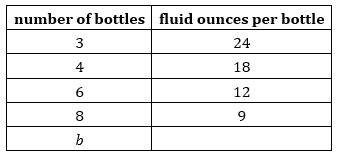# Week 5 Day 2 Lesson Summary

In the lesson, you used a number of ways to reason about the relationship between quantities and to write an equation to represent that relationship. Here is a list of several of those reasoning strategies:
• creating a table to help us see how a quantity changes or how two quantities might be related
• looking for a pattern in the table: noticing how the values in a table change from one row to the next, or from one column to the next
• trying different numbers for one variable and observing how they affect the other variables

A student starts a new semester with \$30 in their lunch account. Each lunch at school costs \$1.75. What's the relationship between the number of school lunches purchased, n, and the dollar amount in the account, A?

A chef is pouring oil from a large jug into equal-size bottles. This table shows the relationship between the number of bottles used and the volume of oil, in fluid ounces, in each bottle. Write an equation to show this relationship.An equation that contains only one unknown quantity or one quantity that can vary is called an equation in one variable.  For example, the equation 2l + 2w = 72 represents the relationship between the length, l, and the width, w, of a rectangle that has a perimeter of 72 units. If we know that the length is 15 units, we can rewrite the equation as: 2(15) + 2w = 72 This is an equation in one variable, because w is the only quantity that we don't know. To solve this equation means to find a value of w that makes the equation true. In this case, 21 is the solution.

An equation that contains two unknown quantities or two quantities that vary is called an equation in two variables. A solution to such an equation is a pair of numbers that makes the equation true.  Suppose Tyler spends \$45 on T-shirts and socks. A T-shirt costs \$10 and a pair of socks costs \$2.50. If t represents the number of T-shirts and p represents the number of pairs of socks that Tyler buys, we can can represent this situation with the equation: 10t + 2.50p = 45 This is an equation in two variables. More than one pair of values for t and p make the equation true. 2 possible solutions: t = 3 and p = 6, because 10(3) + 2.50(6) = 45 t = 4 and p = 2, because 10(4) + 2.50(2) = 45 What is another possible solution?